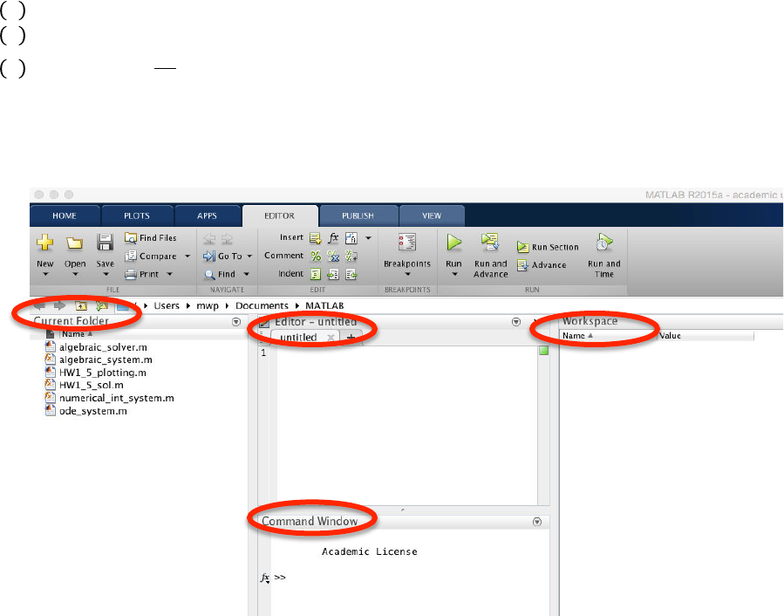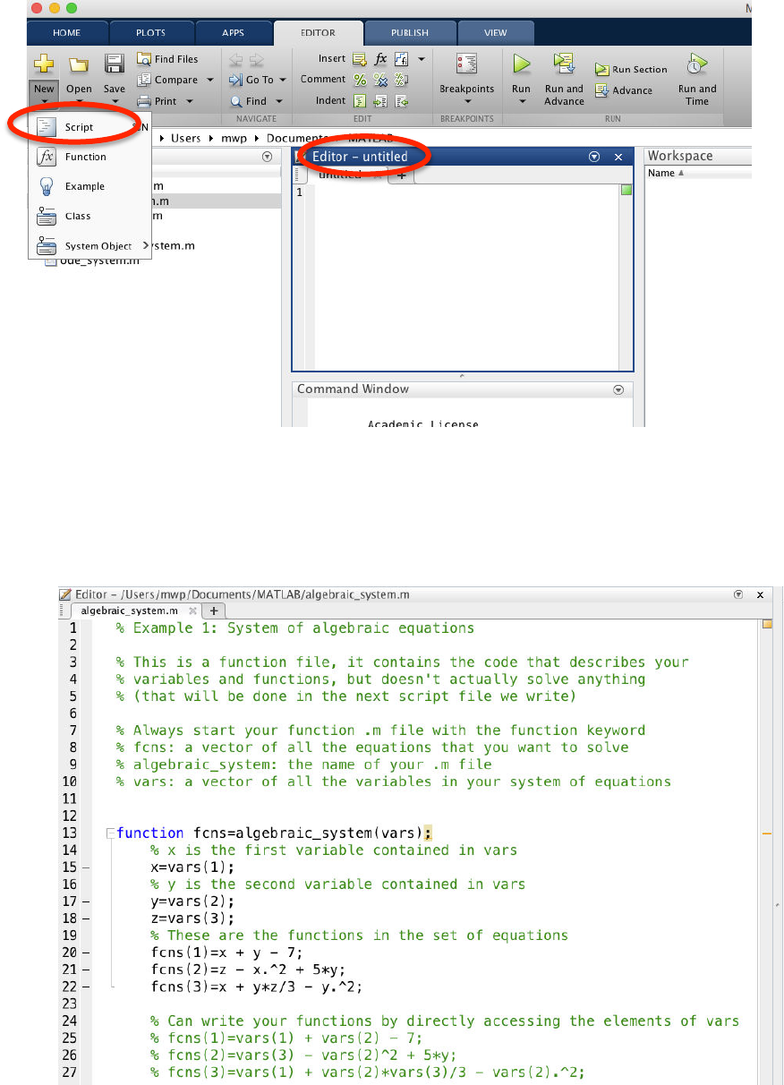# CHM ENG 142 Lecture 3: Matlab Introduction (2)

38 views12 pages
School
Berkeley
Department
Chemical and Biomolecular Engineering
Course
CHM ENG 142
Professor
Matlab Introduction
CBE 142 Fall 2015
This is a brief introduction to MATLAB for CBE 142. Matlab is a powerful numerics
package with diverse capabilities in math and engineering. It is very helpful in a reaction
engineering class because we are often tasked with solving systems of equations, which
can often be quite complex (for example in a coupled, non-linear system of ODEs or
PDE’s describing combined diffusion and reaction). We’ll cover three topics in this
introduction:
(1) Solving a system of algebraic equations
(2) Solving a system of ODEs
(3) Numerical integration
This will give you the general flavor for how to use Matlab to solve numerical problems
and familiarize yourself with the software. In the first example we will give a step-by-
step explanation of how to setup the scripts and run them. In examples two and three we
will just provide .m files with the solutions to the problem, since these can be set up and
executed exactly the same way as described in Example 1. The examples are good
starting points for later in the course when you will need to solve problems numerically.
NOTE: At Berkeley we have an institution license for MATLAB so it’s free to everyone
affiliated with the university, but in reality this software package is very expensive. An
alternative for those who are curious is to check out Python’s SciPy package. If you
know a little programming or can become familiar with the Python language, many of the
mathematical tools in Matlab can be found in SciPy, and the best part is that it’s
completely free to anyone. A great skill to have is some basic working knowledge of a
scientific programming language and this is something a lot of employers might
appreciate, especially if they don’t have a Matlab license.
Unlock document

This preview shows pages 1-3 of the document.
Unlock all 12 pages and 3 million more documents.Example 1: Solving a system of algebraic equations
Solve the following system of equations:
1!0=!+!7
2!0=!!!+5!+!
3!0=!!!+!"
3
This is a straightforward task for Matlab’s fsolve(). Follow the steps below to setup the
necessary files to solve the problem.
Step 1: Familiarize yourself with the User Interface
Current Folder: shows all the files that Matlab can find and use during this session (aka
all the files in your current working directory). For me you can see it’s Users->mwp-
>Documents->MATLAB
Editor: Where you can edit .m files (Matlab script files), the files where your code goes
Command Window: If you don’t want to put your code in script file, you can execute
commands from the Command Window as well. Whether you execute a script or run
your commands manually from the Command Window, the output of your calculations
will go to the Command Window unless you instruct Matlab to do something special
Workspace: This will display all the variables that have been initialized during your
current session.
Unlock document

This preview shows pages 1-3 of the document.
Unlock all 12 pages and 3 million more documents.Step 2: Create script files (.m) to solve the problem of interest
Under the editor tab, choose new script file, and the blank script file will appear in the
Editor window.
Step 3: Write the code for a function that holds your system of equations in this script file
Save the file as algebraic_system.m and you should see it appear in the Current Folder
window. Then enter the text shown in the example below. The comments explain how
the code works.
Unlock document

This preview shows pages 1-3 of the document.
Unlock all 12 pages and 3 million more documents.

## Document Summary

This is a brief introduction to matlab for cbe 142. Matlab is a powerful numerics package with diverse capabilities in math and engineering. It is very helpful in a reaction engineering class because we are often tasked with solving systems of equations, which can often be quite complex (for example in a coupled, non-linear system of odes or. We"ll cover three topics in this introduction: (1) solving a system of algebraic equations (2) solving a system of odes (3) numerical integration. This will give you the general flavor for how to use matlab to solve numerical problems and familiarize yourself with the software. In the first example we will give a step-by- step explanation of how to setup the scripts and run them. In examples two and three we will just provide . m files with the solutions to the problem, since these can be set up and executed exactly the same way as described in example 1.